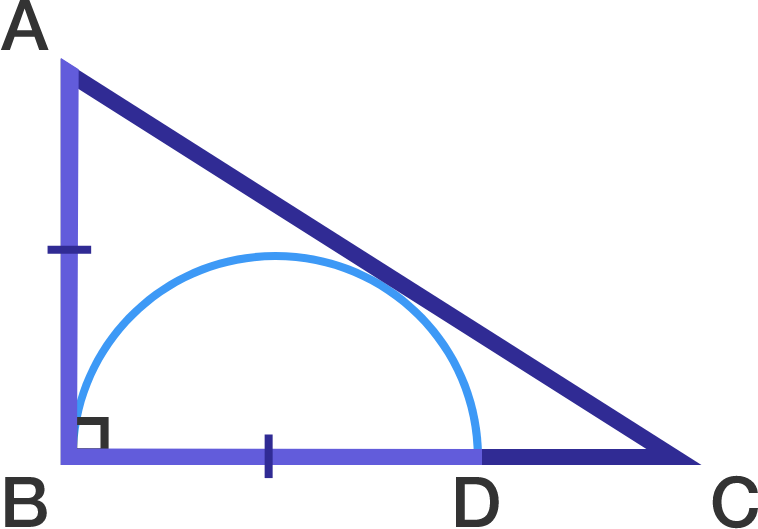# Day 18: An Incredible Result

Geometry Level 4A triangle $ABC$ has a right angle at $B$. A semicircle is inscribed within on the side $BC$ and its diameter is equal to $AB$.

If the ratio $\frac{AB}{BC}$ can be written in the form $\frac{a}{b}$ where $a$ and $b$ are positive coprime integers, find $a+b$.

This problem is part of the set Advent Calendar 2014.

×